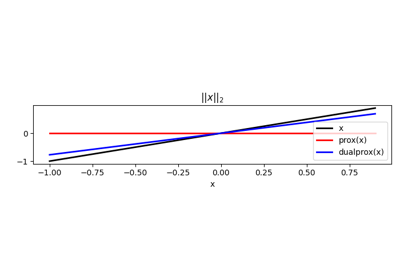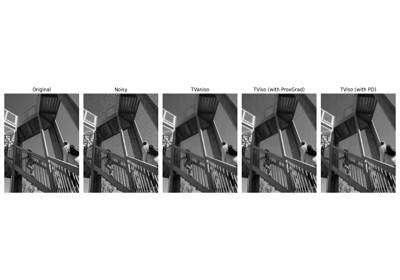# pyproximal.TV#

class pyproximal.TV(dims, sigma=1.0, niter=10, rtol=0.0001, **kwargs)[source]#

TV Norm proximal operator.

Proximal operator for the TV norm defined as: $$f(\mathbf{x}) = \sigma ||\mathbf{x}||_{\text{TV}}$$.

Parameters
dimstuple

Number of samples for each dimension (None if only one dimension is available)

sigmaint, optional

Multiplicative coefficient of TV norm

niterint or func, optional

Number of iterations of iterative scheme used to compute the proximal. This can be a constant number or a function that is called passing a counter which keeps track of how many times the prox method has been invoked before and returns the niter to be used.

rtolfloat, optional

Relative tolerance for stopping criterion.

Notes

The proximal algorithm is implemented following .

1

Beck, A. and Teboulle, M., “Fast gradient-based algorithms for constrained total variation image denoising and deblurring problems”, 2009.

Methods

 __init__(dims[, sigma, niter, rtol]) affine_addition(v) Affine addition chain(g) Chain grad(x) Compute gradient postcomposition(sigma) Postcomposition precomposition(a, b) Precomposition prox(*args, **kwargs) proxdual(**kwargs)

## Examples using pyproximal.TV#Norms

NormsDenoising

Denoising